Скачать презентацию Bivariate Hotspot Detection The circle-based Sa TScan and

• Количество слайдов: 23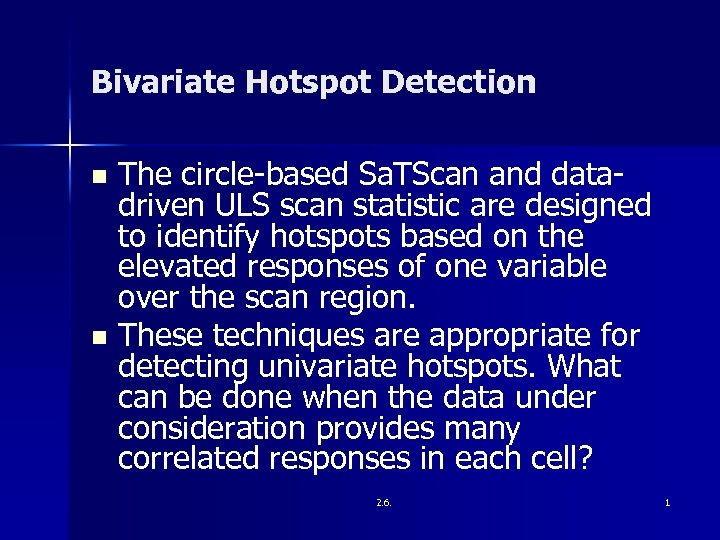Bivariate Hotspot Detection The circle-based Sa. TScan and datadriven ULS scan statistic are designed to identify hotspots based on the elevated responses of one variable over the scan region. n These techniques are appropriate for detecting univariate hotspots. What can be done when the data under consideration provides many correlated responses in each cell? n 2. 6. 1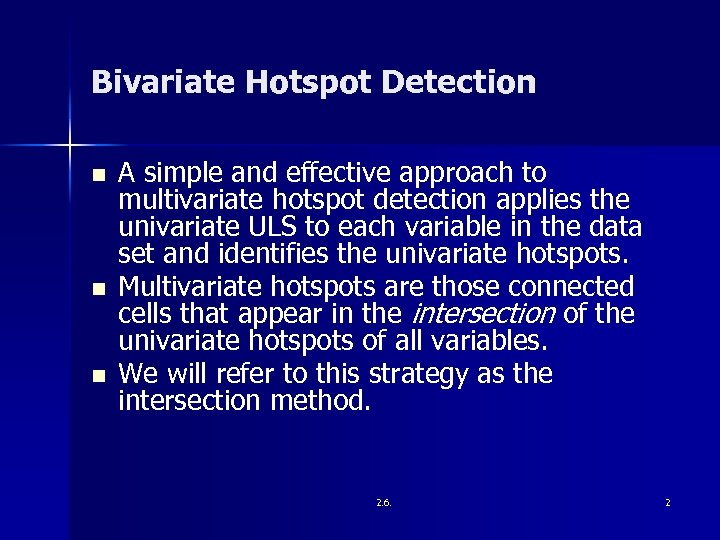Bivariate Hotspot Detection n A simple and effective approach to multivariate hotspot detection applies the univariate ULS to each variable in the data set and identifies the univariate hotspots. Multivariate hotspots are those connected cells that appear in the intersection of the univariate hotspots of all variables. We will refer to this strategy as the intersection method. 2. 6. 2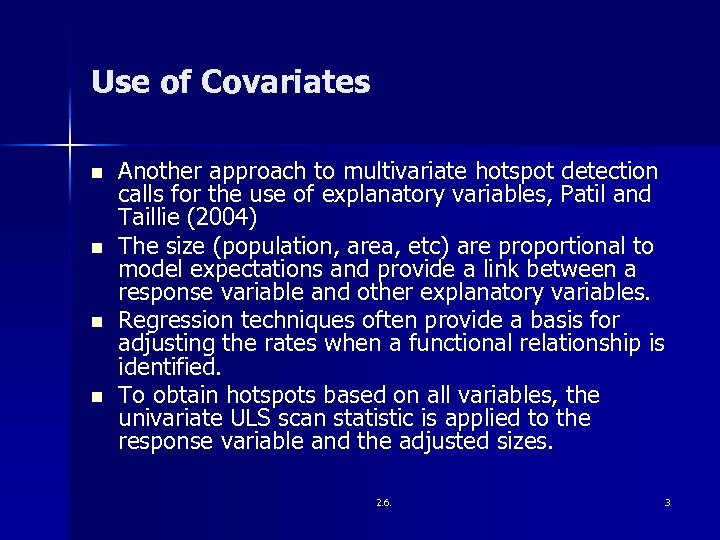Use of Covariates n n Another approach to multivariate hotspot detection calls for the use of explanatory variables, Patil and Taillie (2004) The size (population, area, etc) are proportional to model expectations and provide a link between a response variable and other explanatory variables. Regression techniques often provide a basis for adjusting the rates when a functional relationship is identified. To obtain hotspots based on all variables, the univariate ULS scan statistic is applied to the response variable and the adjusted sizes. 2. 6. 3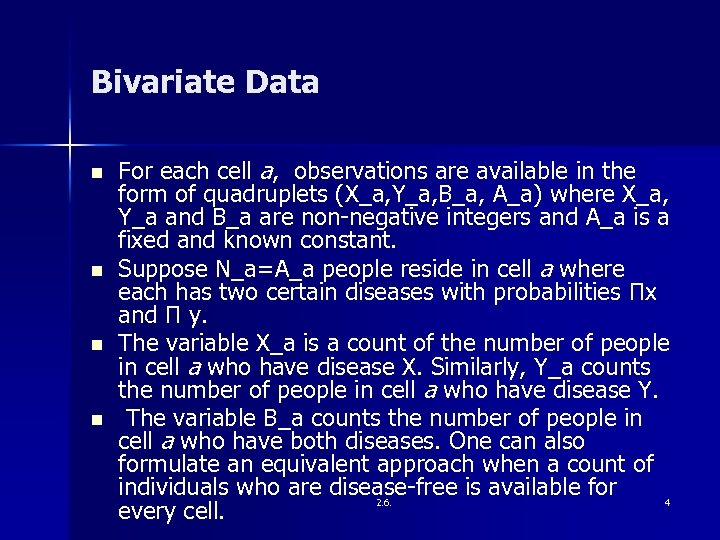Bivariate Data n n For each cell a, observations are available in the form of quadruplets (X_a, Y_a, B_a, A_a) where X_a, Y_a and B_a are non-negative integers and A_a is a fixed and known constant. Suppose N_a=A_a people reside in cell a where each has two certain diseases with probabilities Πx and Π y. The variable X_a is a count of the number of people in cell a who have disease X. Similarly, Y_a counts the number of people in cell a who have disease Y. The variable B_a counts the number of people in cell a who have both diseases. One can also formulate an equivalent approach when a count of individuals who are disease-free is available for 2. 6. 4 every cell.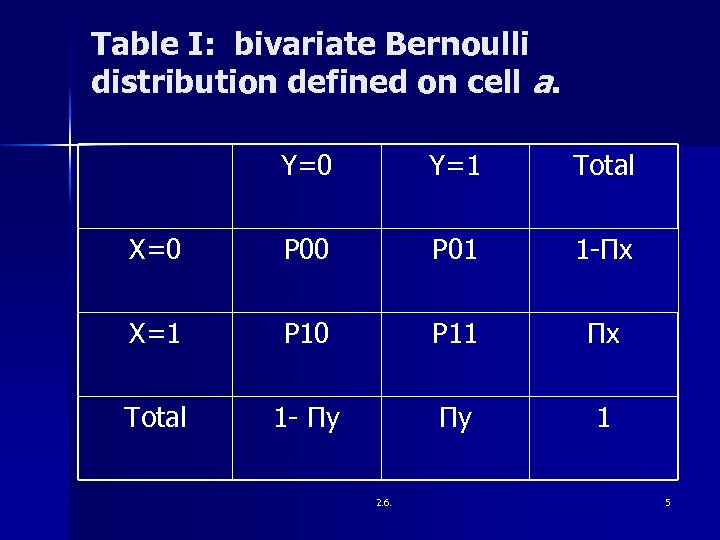Table I: bivariate Bernoulli distribution defined on cell a. Y=0 Y=1 Total X=0 P 01 1 -Πx X=1 P 10 P 11 Πx Total 1 - Πy Πy 1 2. 6. 5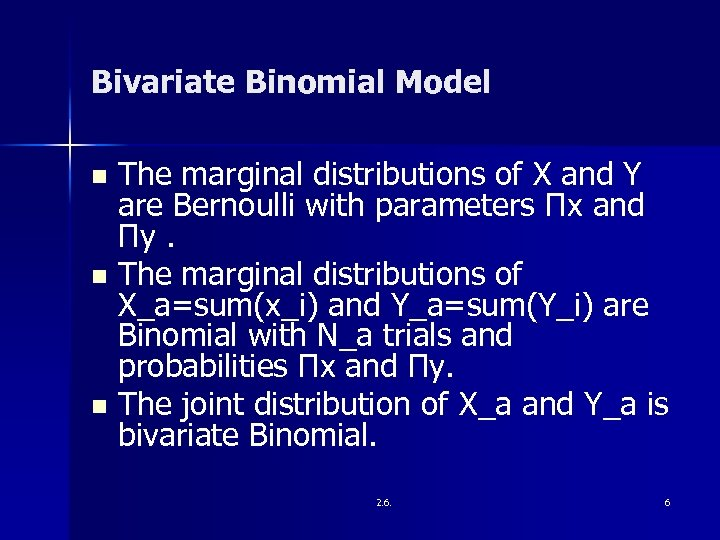Bivariate Binomial Model The marginal distributions of X and Y are Bernoulli with parameters Πx and Πy. n The marginal distributions of X_a=sum(x_i) and Y_a=sum(Y_i) are Binomial with N_a trials and probabilities Πx and Πy. n The joint distribution of X_a and Y_a is bivariate Binomial. n 2. 6. 6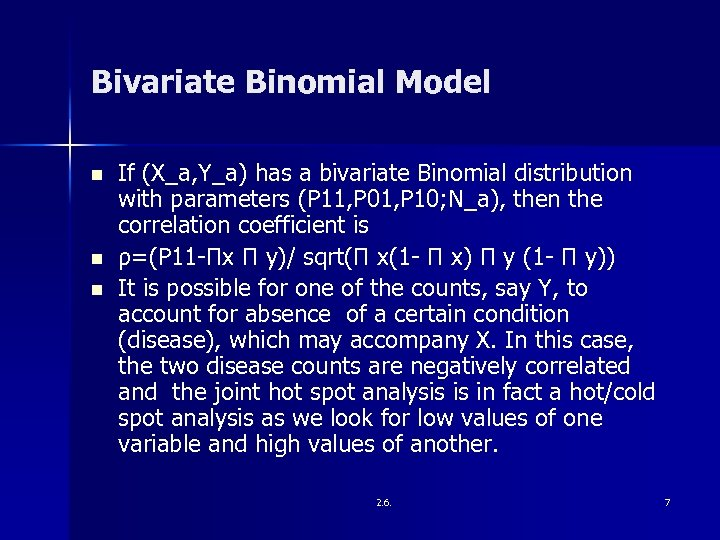Bivariate Binomial Model n n n If (X_a, Y_a) has a bivariate Binomial distribution with parameters (P 11, P 01, P 10; N_a), then the correlation coefficient is ρ=(P 11 -Πx Π y)/ sqrt(Π x(1 - Π x) Π y (1 - Π y)) It is possible for one of the counts, say Y, to account for absence of a certain condition (disease), which may accompany X. In this case, the two disease counts are negatively correlated and the joint hot spot analysis is in fact a hot/cold spot analysis as we look for low values of one variable and high values of another. 2. 6. 7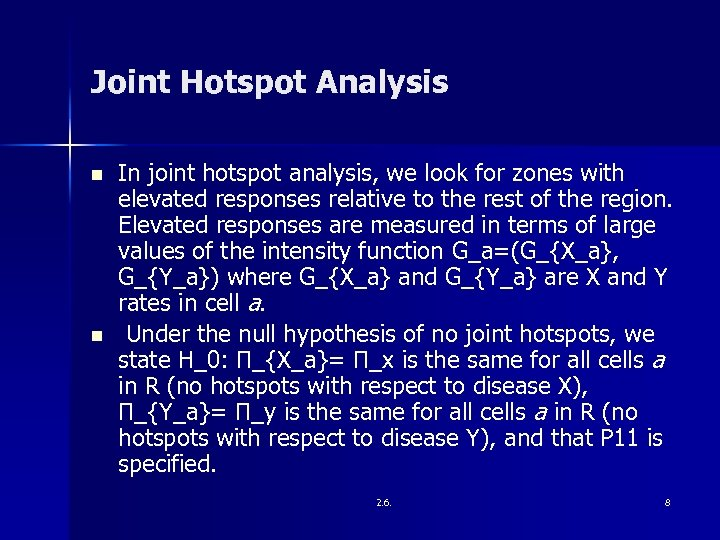Joint Hotspot Analysis n n In joint hotspot analysis, we look for zones with elevated responses relative to the rest of the region. Elevated responses are measured in terms of large values of the intensity function G_a=(G_{X_a}, G_{Y_a}) where G_{X_a} and G_{Y_a} are X and Y rates in cell a. Under the null hypothesis of no joint hotspots, we state H_0: Π_{X_a}= Π_x is the same for all cells a in R (no hotspots with respect to disease X), Π_{Y_a}= Π_y is the same for all cells a in R (no hotspots with respect to disease Y), and that P 11 is specified. 2. 6. 8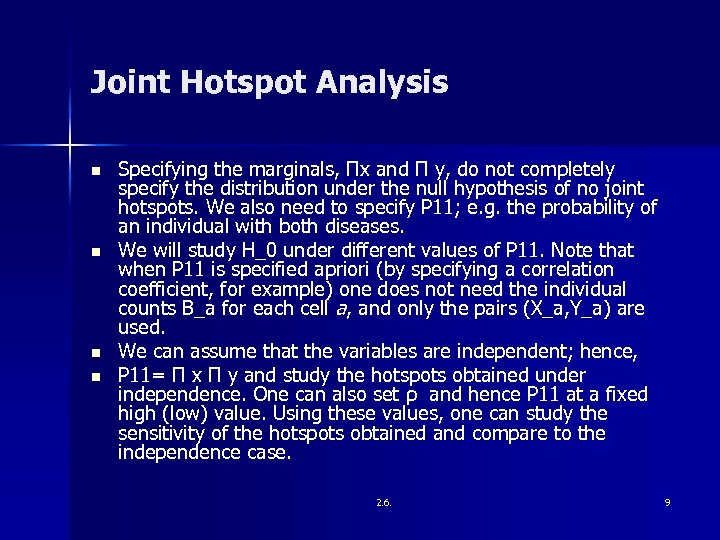Joint Hotspot Analysis n n Specifying the marginals, Πx and Π y, do not completely specify the distribution under the null hypothesis of no joint hotspots. We also need to specify P 11; e. g. the probability of an individual with both diseases. We will study H_0 under different values of P 11. Note that when P 11 is specified apriori (by specifying a correlation coefficient, for example) one does not need the individual counts B_a for each cell a, and only the pairs (X_a, Y_a) are used. We can assume that the variables are independent; hence, P 11= Π x Π y and study the hotspots obtained under independence. One can also set ρ and hence P 11 at a fixed high (low) value. Using these values, one can study the sensitivity of the hotspots obtained and compare to the independence case. 2. 6. 9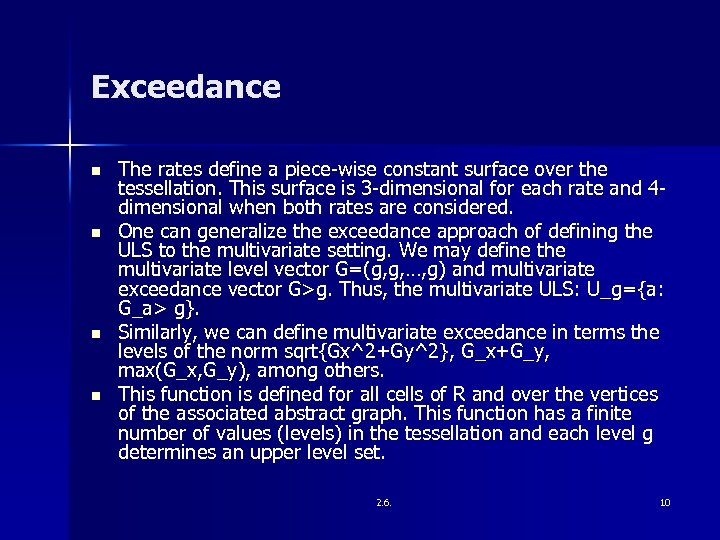Exceedance n n The rates define a piece-wise constant surface over the tessellation. This surface is 3 -dimensional for each rate and 4 dimensional when both rates are considered. One can generalize the exceedance approach of defining the ULS to the multivariate setting. We may define the multivariate level vector G=(g, g, …, g) and multivariate exceedance vector G>g. Thus, the multivariate ULS: U_g={a: G_a> g}. Similarly, we can define multivariate exceedance in terms the levels of the norm sqrt{Gx^2+Gy^2}, G_x+G_y, max(G_x, G_y), among others. This function is defined for all cells of R and over the vertices of the associated abstract graph. This function has a finite number of values (levels) in the tessellation and each level g determines an upper level set. 2. 6. 10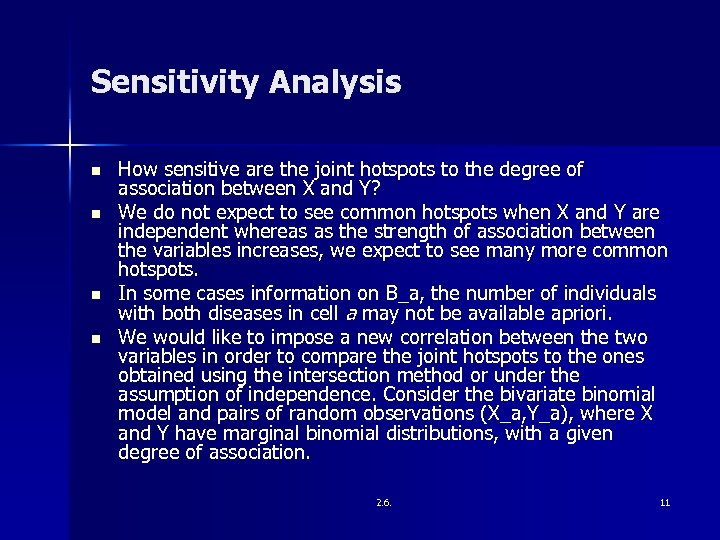Sensitivity Analysis n n How sensitive are the joint hotspots to the degree of association between X and Y? We do not expect to see common hotspots when X and Y are independent whereas as the strength of association between the variables increases, we expect to see many more common hotspots. In some cases information on B_a, the number of individuals with both diseases in cell a may not be available apriori. We would like to impose a new correlation between the two variables in order to compare the joint hotspots to the ones obtained using the intersection method or under the assumption of independence. Consider the bivariate binomial model and pairs of random observations (X_a, Y_a), where X and Y have marginal binomial distributions, with a given degree of association. 2. 6. 11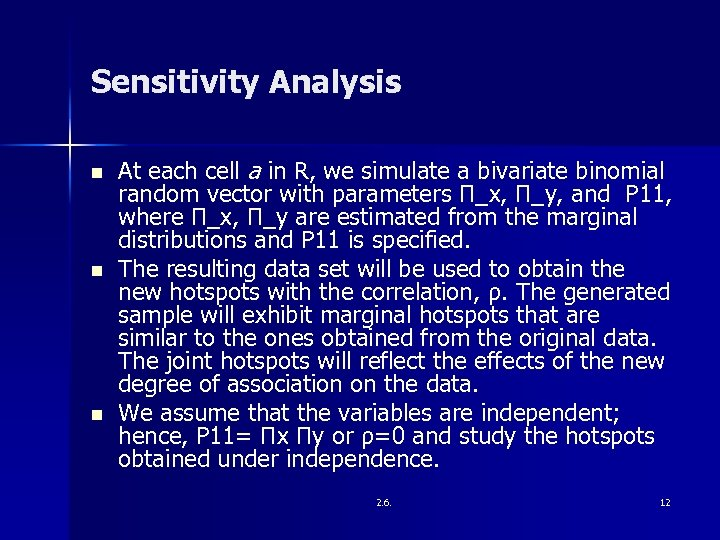Sensitivity Analysis n n n At each cell a in R, we simulate a bivariate binomial random vector with parameters Π_x, Π_y, and P 11, where Π_x, Π_y are estimated from the marginal distributions and P 11 is specified. The resulting data set will be used to obtain the new hotspots with the correlation, ρ. The generated sample will exhibit marginal hotspots that are similar to the ones obtained from the original data. The joint hotspots will reflect the effects of the new degree of association on the data. We assume that the variables are independent; hence, P 11= Πx Πy or ρ=0 and study the hotspots obtained under independence. 2. 6. 12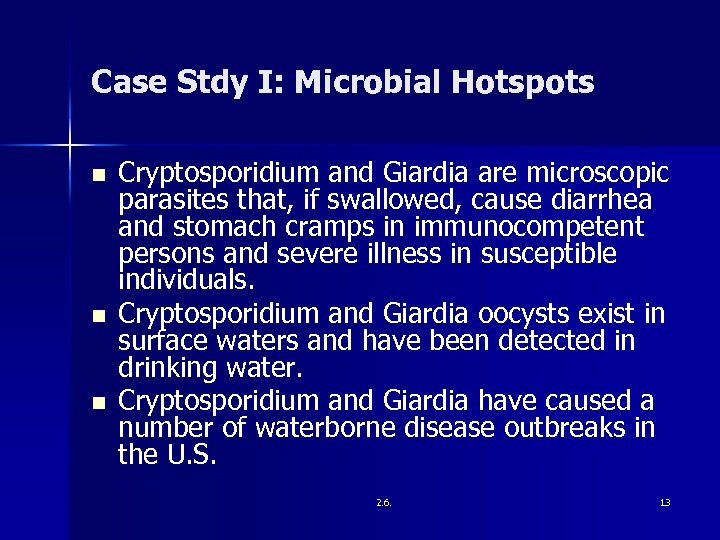Case Stdy I: Microbial Hotspots n n n Cryptosporidium and Giardia are microscopic parasites that, if swallowed, cause diarrhea and stomach cramps in immunocompetent persons and severe illness in susceptible individuals. Cryptosporidium and Giardia oocysts exist in surface waters and have been detected in drinking water. Cryptosporidium and Giardia have caused a number of waterborne disease outbreaks in the U. S. 2. 6. 13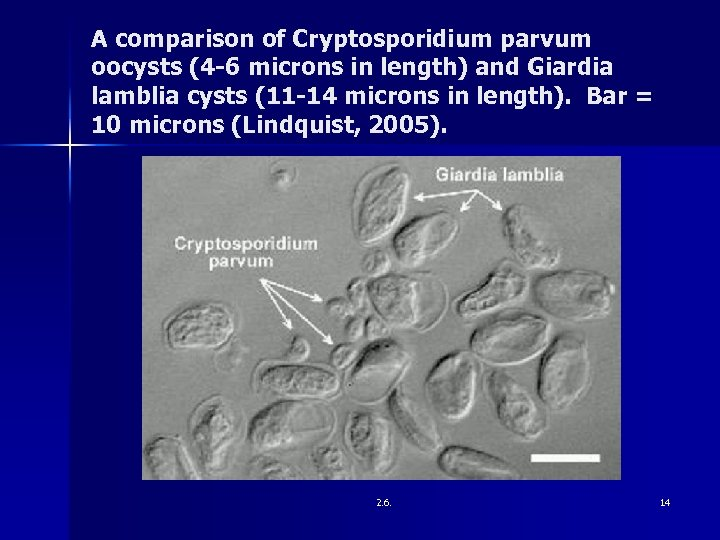A comparison of Cryptosporidium parvum oocysts (4 -6 microns in length) and Giardia lamblia cysts (11 -14 microns in length). Bar = 10 microns (Lindquist, 2005). 2. 6. 14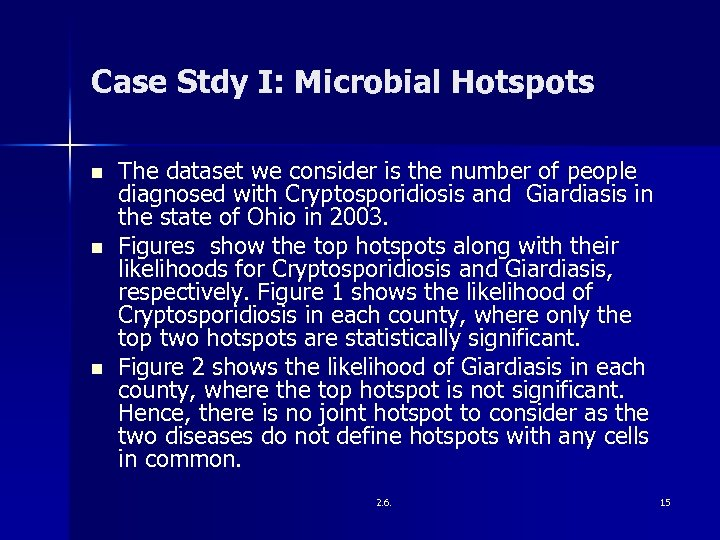Case Stdy I: Microbial Hotspots n n n The dataset we consider is the number of people diagnosed with Cryptosporidiosis and Giardiasis in the state of Ohio in 2003. Figures show the top hotspots along with their likelihoods for Cryptosporidiosis and Giardiasis, respectively. Figure 1 shows the likelihood of Cryptosporidiosis in each county, where only the top two hotspots are statistically significant. Figure 2 shows the likelihood of Giardiasis in each county, where the top hotspot is not significant. Hence, there is no joint hotspot to consider as the two diseases do not define hotspots with any cells in common. 2. 6. 15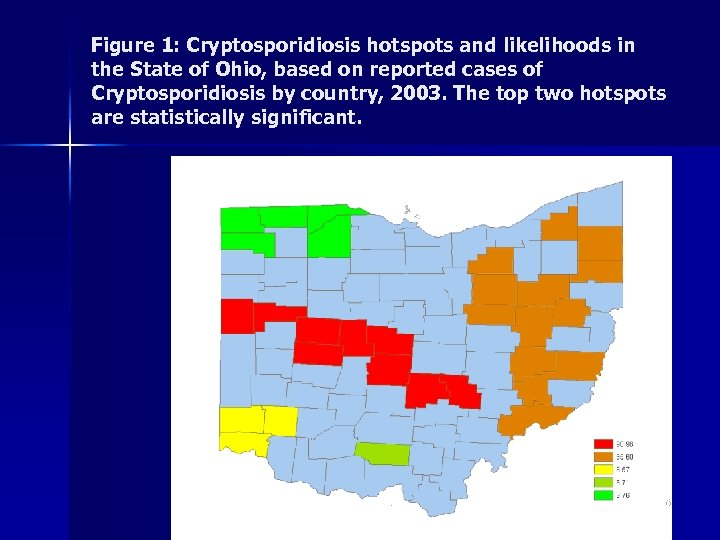Figure 1: Cryptosporidiosis hotspots and likelihoods in the State of Ohio, based on reported cases of Cryptosporidiosis by country, 2003. The top two hotspots are statistically significant. 2. 6. 16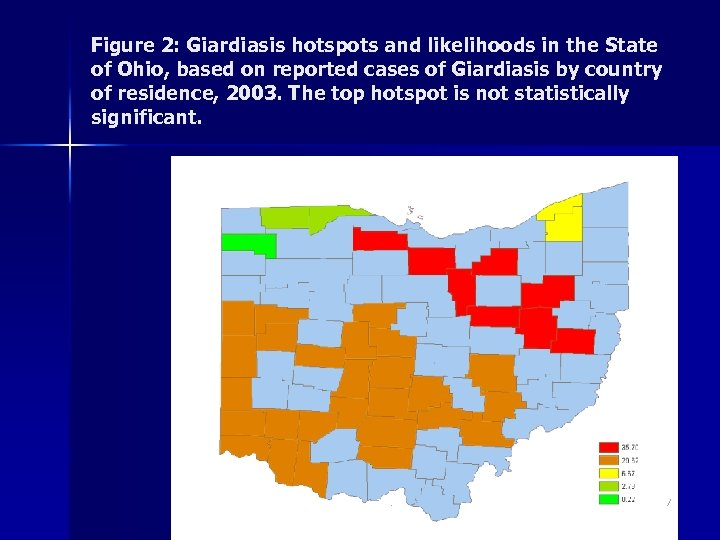Figure 2: Giardiasis hotspots and likelihoods in the State of Ohio, based on reported cases of Giardiasis by country of residence, 2003. The top hotspot is not statistically significant. 2. 6. 17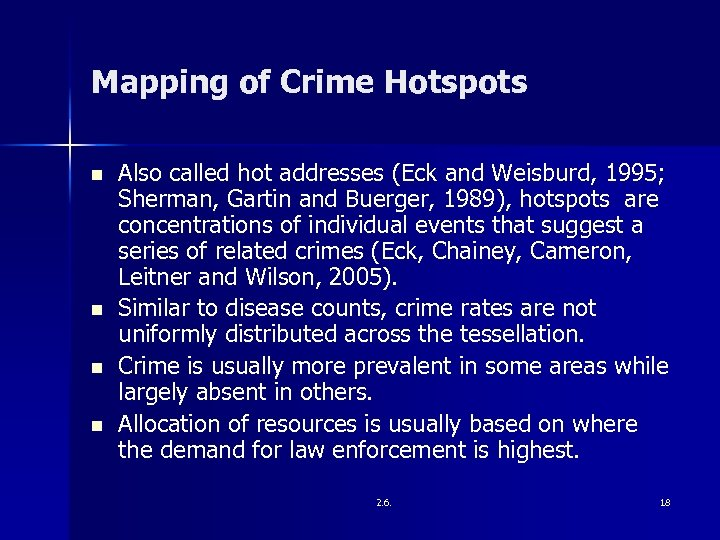Mapping of Crime Hotspots n n Also called hot addresses (Eck and Weisburd, 1995; Sherman, Gartin and Buerger, 1989), hotspots are concentrations of individual events that suggest a series of related crimes (Eck, Chainey, Cameron, Leitner and Wilson, 2005). Similar to disease counts, crime rates are not uniformly distributed across the tessellation. Crime is usually more prevalent in some areas while largely absent in others. Allocation of resources is usually based on where the demand for law enforcement is highest. 2. 6. 18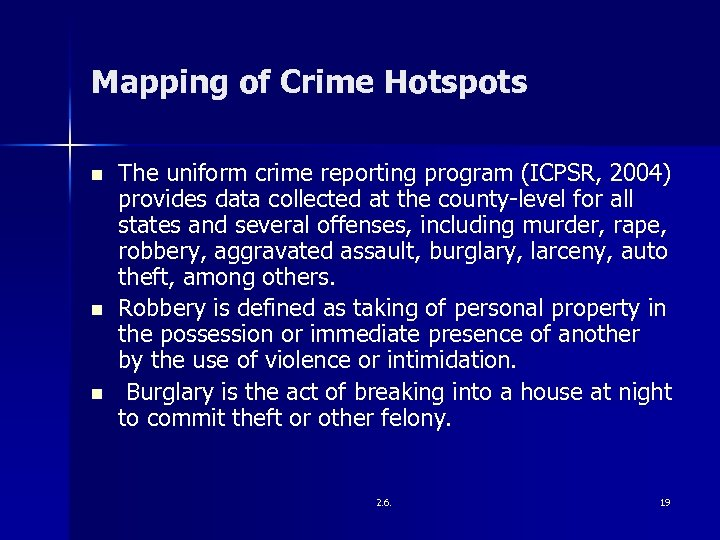Mapping of Crime Hotspots n n n The uniform crime reporting program (ICPSR, 2004) provides data collected at the county-level for all states and several offenses, including murder, rape, robbery, aggravated assault, burglary, larceny, auto theft, among others. Robbery is defined as taking of personal property in the possession or immediate presence of another by the use of violence or intimidation. Burglary is the act of breaking into a house at night to commit theft or other felony. 2. 6. 19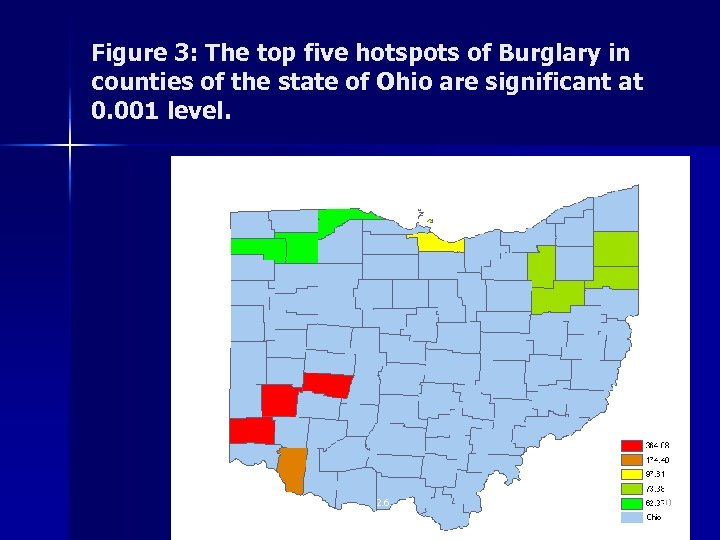Figure 3: The top five hotspots of Burglary in counties of the state of Ohio are significant at 0. 001 level. 2. 6. 20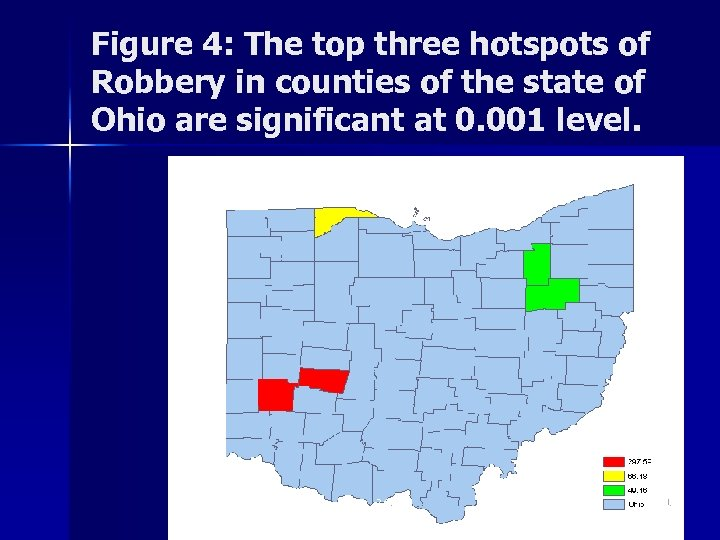Figure 4: The top three hotspots of Robbery in counties of the state of Ohio are significant at 0. 001 level. 2. 6. 21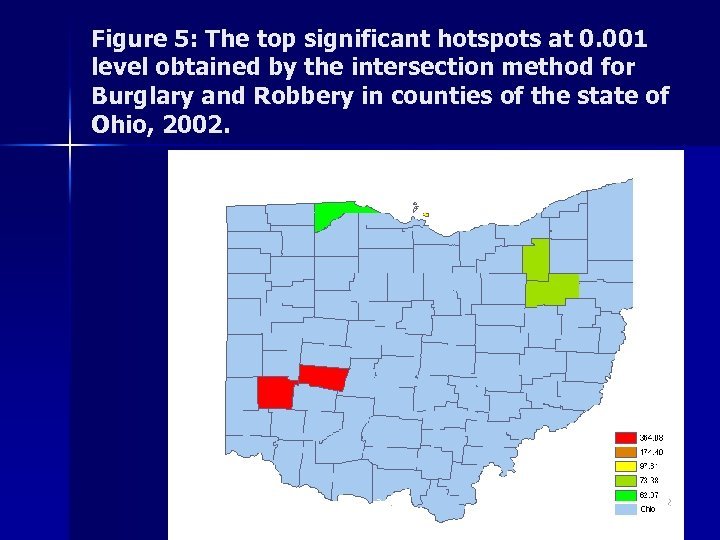Figure 5: The top significant hotspots at 0. 001 level obtained by the intersection method for Burglary and Robbery in counties of the state of Ohio, 2002. 2. 6. 22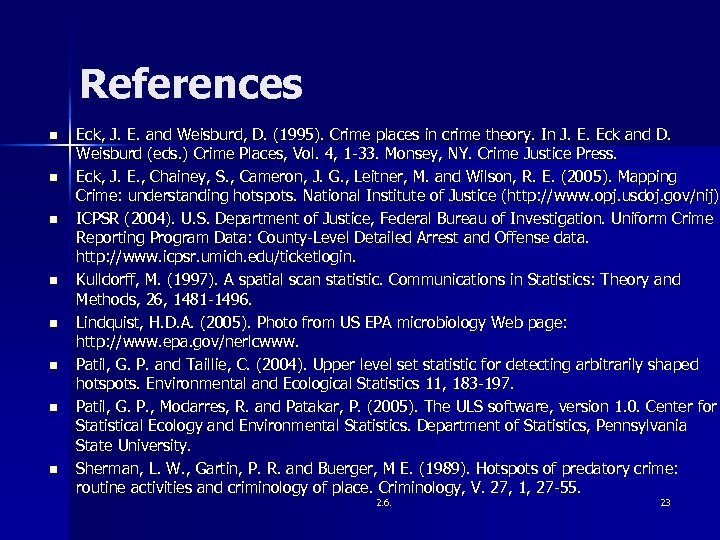References n n n n Eck, J. E. and Weisburd, D. (1995). Crime places in crime theory. In J. E. Eck and D. Weisburd (eds. ) Crime Places, Vol. 4, 1 -33. Monsey, NY. Crime Justice Press. Eck, J. E. , Chainey, S. , Cameron, J. G. , Leitner, M. and Wilson, R. E. (2005). Mapping Crime: understanding hotspots. National Institute of Justice (http: // www. opj. usdoj. gov/nij). ICPSR (2004). U. S. Department of Justice, Federal Bureau of Investigation. Uniform Crime Reporting Program Data: County-Level Detailed Arrest and Offense data. http: //www. icpsr. umich. edu/ticketlogin. Kulldorff, M. (1997). A spatial scan statistic. Communications in Statistics: Theory and Methods, 26, 1481 -1496. Lindquist, H. D. A. (2005). Photo from US EPA microbiology Web page: http: //www. epa. gov/nerlcwww. Patil, G. P. and Taillie, C. (2004). Upper level set statistic for detecting arbitrarily shaped hotspots. Environmental and Ecological Statistics 11, 183 -197. Patil, G. P. , Modarres, R. and Patakar, P. (2005). The ULS software, version 1. 0. Center for Statistical Ecology and Environmental Statistics. Department of Statistics, Pennsylvania State University. Sherman, L. W. , Gartin, P. R. and Buerger, M E. (1989). Hotspots of predatory crime: routine activities and criminology of place. Criminology, V. 27, 1, 27 -55. 2. 6. 23